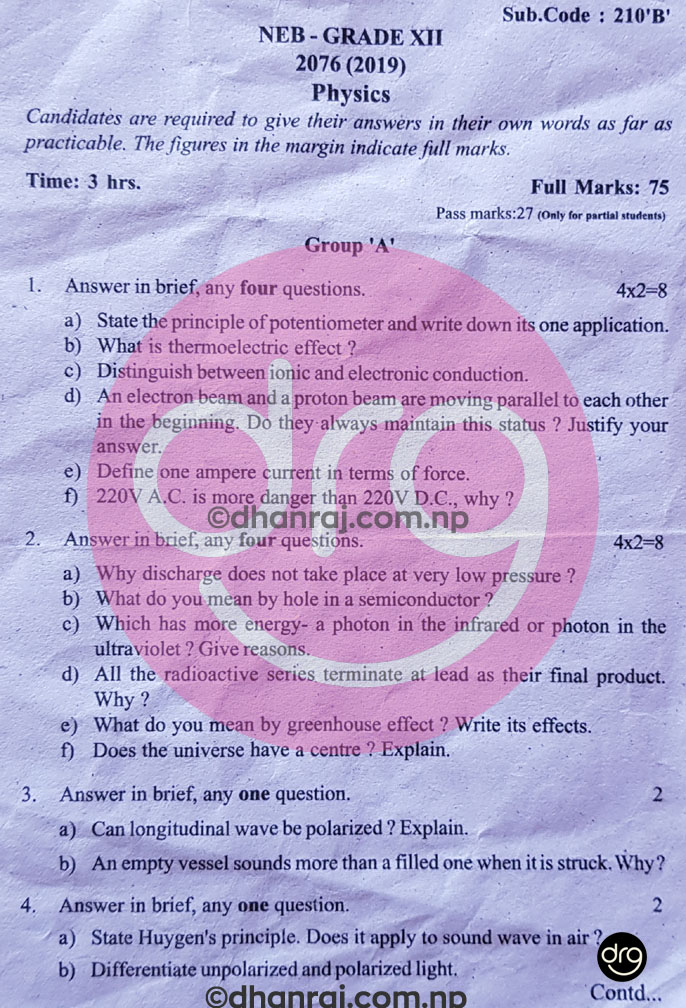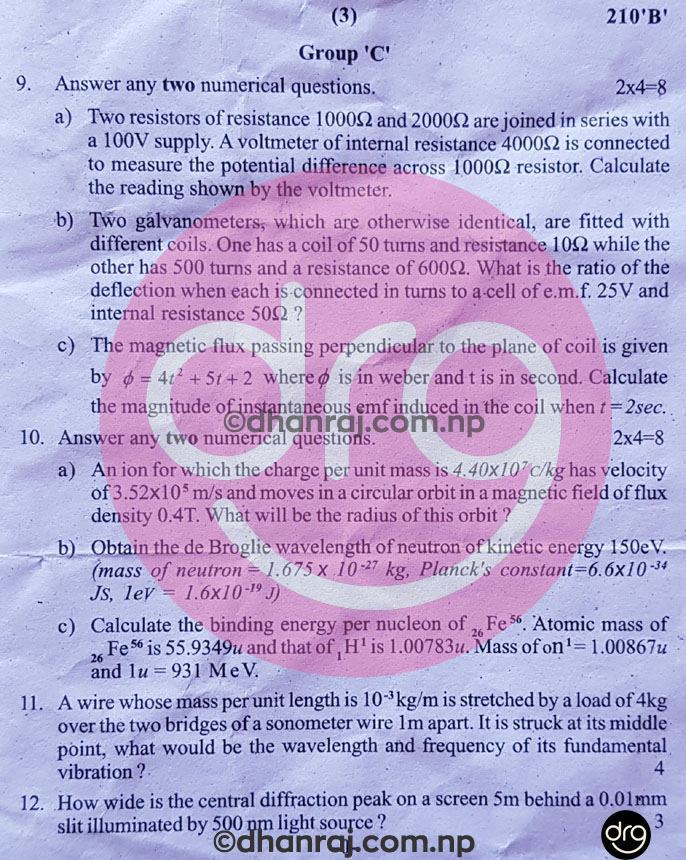# Physics | Grade 12-XII | Question Paper 2076  | National Examinations Board

NATIONAL EXAMINATIONS BOARD [NEB]
Examination - 2076 (2019)
Regular Students | Subject Code: 210 ‘B’
Time - 3 hrs
Full Marks - 75
Pass Marks - 27
Candidates are required to give their answers in their own words as far as practicable. The figures in the margin indicate full marks.

Group 'A'

1. Answer in brief, any four questions: [4x2=8]

a) State the principle of potentiometer and write down its one application.
b) What is a thermoelectric effect?
c) Distinguish between ionic and electronic conduction.
d) An electronic beam and a proton beam are moving parallel to each other in the beginning. Do they always maintain this status? Justify your answer.
e) Define one ampere current in terms of force.
f) 220V A.C. is more danger than 220V D.C., why?

2. Answer in brief, any four questions. [4x2=8]

a) Why discharge does not take place at very low pressure?
b) What do you mean by hole in a semiconductor?
c) Which has more energy - a photon in the infrared or photon in the ultraviolet? Give reasons.
d) All the radioactive series terminate at lead as their final product. Why?
e) What do you mean by greenhouse effect? Write its effects.
f) Does the universe have a centre? Explain.
3. Answer in brief, any one question. 

a) Can longitudinal wave be polarized? Explain.
b) An empty vessel sounds more than a filled one when it is struck. Why?

4. Answer in brief, any one question. 

a) State Huygen's principle. Does it apply to sound wave in air?
b) Differentiate unpolarized and polarized light.

Group 'B'

5. Answer any three questions:  [3x4=12]

a) What do you mean by shunt? Describe its use in converting a galvanometer into an ammeter?
b) State Joule's law of heating and verify it experimentally.
c) State Biot and Savart law. Derive an expression for the magnetic field at a point due to a long straight conductor carrying current.
d) An alternating current passes through a circuit containing an inductor and a resistor in series. Derive expressions for the current flowing and phase relation between the current and the voltage.

6. Answer any three questions: [3x4=12]

a) What is quantization of charge? Describe the theory of Millikan's oil drop experiment to determine the number of charges on an oil drop.
b) What is P-N junction diode? Discuss its applications as full wave rectifier.
c) List out the laws of radioactive disintegration. Deduce the expression N= where symbols have their usual meaning.
d) What are sources of energy? Discuss global energy consumption pattern and demands.

7. Answer any one question: 

a) Does the propagation of sound wave cause change in thermodynamic condition of medium? Derive Laplace formula of velocity of sound in air.
b) What is Doppler's effect? Derive an expression for the apparent frequency received by a stationary observer when a source of sound is moving away from the observer.

8. Answer any one question: 

a) Describe Newton's ring experiment and derive expression for wavelength of light.
b) Describe Foucault's method of determining the speed of light.
Group 'C'

9. Answer any two question: [2x4=8]

a) Two resistors of resistance 1000Ω are joined in series with a 100V supply. A voltmeter of internal resistance 4000Ω is connected to measure the potential difference across 1000Ω resistor. Calculate the reading shown by the voltmeter.
b) Two galvanometers, which are otherwise identical, are fitted with different coils. One has a coil of 50 turns and resistance 10Ω while the other has 500 turns and resistance of 600Ω. What is the ratio of the deflection when each is connected in turns to a cell of e.m.f. 25V and internal resistance 50Ω?
c) The magnetic flux passing perpendicular to the plane of coil is given by ϕ = 4t2 +5t + 2 where ϕ is in weber and t is in second. Calculate the magnitude of instantaneous emf induced in the coil when t=2sec.

10. Answer any two question: [2x4=8]

a) An ion for which the charge per unit mass is 4.40x10c/kg has velocity of 3.52x10m/s and moves in a circular orbit in a magnetic field of flux density 0.4T. What will be the radius of this orbit?
b) Obtain the de Broglie wavelength of neutron of kinetic energy 150eV. (mass of neutron = 1.675 x 10-27 kg, Planck's constant = 6.6 x 10-34 JS, 1eV = 1.6x10-19 J)
c) Calculate the binding energy per nucleon of 26Fe56. Atomic mass of 26Fe56 is 55.9349u and that of 1H1 is 1.00783u. Mass of on= 1.00867u and 1u = 931 MeV.

11. A wire whose mass per unit length is 10-3 kg/m is stretched by a load of 4kg over the two bridges of a sonometer wire 1m apart. It is struck at its middle point, what would be the wavelength and frequency of its fundamental vibration? 

12. How wide is the central diffraction peak on a screen 5m behind a 0.01mm slit illuminated by 500 nm light source? 

View/see Grade XII-12 Question Paper of Physics 2076/2019 NEB:Physics-Grade 12-XII-Question-Paper-2076-2019-NEBPhysics-Grade 12-XII-Question-Paper-2076-2019-NEBPhysics-Grade 12-XII-Question-Paper-2076-2019-NEB

### 2 Responses to "Physics | Grade 12-XII | Question Paper 2076  | National Examinations Board"

1.1.Dear Dinesh,